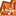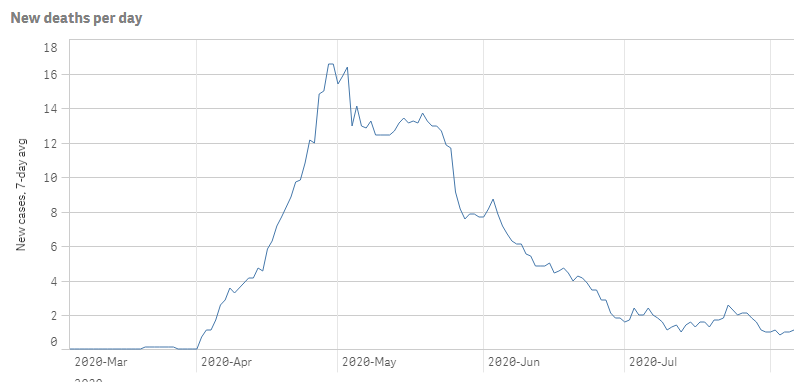# App Development

Announcements
Read about the latest Qlik Community enhancements on the Community News blog!
cancel
Showing results for
Did you mean:Contributor

## Set Analysis on complex expression

Hi all, I created an app linked to the NYT GitHub covid data by county, which shows total cases and deaths per day.  I created a couple combo charts with modifiers so that I could figure out how to get daily case totals and then a 7-day moving average of daily case totals.

I'm trying to use set analysis to chart specific fips codes so I can plot multiple counties' 7-day averages on one chart.  Once I used the modifiers for difference and then moving average, I copied and pasted the "output expressions" to create new charts with them.  The 7-day average for this data is the expression below:

RangeSum(Above(If(Count([\$(=Replace(GetObjectField(0),']',']]'))]) > 0, (　If(Count([\$(=Replace(GetObjectField(0),']',']]'))]) > 0, (　Sum(cases)　) + Sum({1<[\$(=Replace(GetObjectField(0),']',']]'))]={">=\$(=Min([\$(=Replace(GetObjectField(0),']',']]'))]))<=\$(=Max([\$(=Replace(GetObjectField(0),']',']]'))]))"}>}0), 0) - Above(If(Count([\$(=Replace(GetObjectField(0),']',']]'))]) > 0, (　Sum(cases)　) + Sum({1<[\$(=Replace(GetObjectField(0),']',']]'))]={">=\$(=Min([\$(=Replace(GetObjectField(0),']',']]'))]))<=\$(=Max([\$(=Replace(GetObjectField(0),']',']]'))]))"}>}0), 0))　) + Sum({1<[\$(=Replace(GetObjectField(0),']',']]'))]={">=\$(=Min([\$(=Replace(GetObjectField(0),']',']]'))]))<=\$(=Max([\$(=Replace(GetObjectField(0),']',']]'))]))"}>}0), 0), 0, 7)) / RangeMin(7, RowNo())

The field I want to use set analysis for is "fips" and an example fips code I want to use is "24031".  Let me know if you have any ideas how to use set analysis on this expression!  I have tried nesting it in different locations in the expression to no avail.

Labels (1)
• ### Qlik Sense

1 Solution

Accepted SolutionsMVP

Is this what you want?``````RangeSum(Above(If(Count({\$<fips={'24031'}>}[\$(=Replace(GetObjectField(0),']',']]'))])>0,
(　If(Count({\$<fips={'24031'}>}[\$(=Replace(GetObjectField(0),']',']]'))])>0,
(　Sum({\$<fips={'24031'}>}deaths)　)+Sum({1<fips={'24031'}, [\$(=Replace(GetObjectField(0),']',']]'))]={">=\$(=Min([\$(=Replace(GetObjectField(0),']',']]'))]))<=\$(=Max([\$(=Replace(GetObjectField(0),']',']]'))]))"}>}0),0)-Above(If(Count([\$(=Replace(GetObjectField(0),']',']]'))])>0,
(　Sum({\$<fips={'24031'}>}deaths)　)+Sum({1<fips={'24031'}, [\$(=Replace(GetObjectField(0),']',']]'))]={">=\$(=Min([\$(=Replace(GetObjectField(0),']',']]'))]))<=\$(=Max([\$(=Replace(GetObjectField(0),']',']]'))]))"}>}0),0))　)+Sum({1<[\$(=Replace(GetObjectField(0),']',']]'))]={">=\$(=Min([\$(=Replace(GetObjectField(0),']',']]'))]))<=\$(=Max([\$(=Replace(GetObjectField(0),']',']]'))]))"}>}0),0),0,7))
/RangeMin(7,RowNo())``````

7 RepliesMVP

So, are you saying that in addition to the above expression are you also looking to add set analysis for fips?Contributor
Author

Yes, that expression is what I would like to use as the sum, and I want to separate it by counties (that is, by fips codes).

The set analysis would look something like this in a simpler expression:

Sum({\$<fips={'24031'}>} [7-day average cases])

But the 7-day case average is the entire complex expression above.Contributor
Author

Hi, any ideas on creating this set expression?
Or perhaps a way to make a “difference” and “moving average” field via the load script for a field that reports totals through xx date? That would potentially be easier to apply set analysis; I just don’t know how to do it.MVP

Would you be able to share a sample where we can see the issue?Contributor
Author

Yes, please see the attached qvf.  I'm trying to add the set analysis to the two charts on the third sheet, Counties Daily, using the fips codes in the table on that sheet (when default bookmark is applied).  Does that help show what I'm trying to see?  The two fields are cases and deaths that are being summed by fips code.MVP

Is this what you want?``````RangeSum(Above(If(Count({\$<fips={'24031'}>}[\$(=Replace(GetObjectField(0),']',']]'))])>0,
(　If(Count({\$<fips={'24031'}>}[\$(=Replace(GetObjectField(0),']',']]'))])>0,
(　Sum({\$<fips={'24031'}>}deaths)　)+Sum({1<fips={'24031'}, [\$(=Replace(GetObjectField(0),']',']]'))]={">=\$(=Min([\$(=Replace(GetObjectField(0),']',']]'))]))<=\$(=Max([\$(=Replace(GetObjectField(0),']',']]'))]))"}>}0),0)-Above(If(Count([\$(=Replace(GetObjectField(0),']',']]'))])>0,
(　Sum({\$<fips={'24031'}>}deaths)　)+Sum({1<fips={'24031'}, [\$(=Replace(GetObjectField(0),']',']]'))]={">=\$(=Min([\$(=Replace(GetObjectField(0),']',']]'))]))<=\$(=Max([\$(=Replace(GetObjectField(0),']',']]'))]))"}>}0),0))　)+Sum({1<[\$(=Replace(GetObjectField(0),']',']]'))]={">=\$(=Min([\$(=Replace(GetObjectField(0),']',']]'))]))<=\$(=Max([\$(=Replace(GetObjectField(0),']',']]'))]))"}>}0),0),0,7))
/RangeMin(7,RowNo())``````Contributor
Author

That's perfect, thank you so much!Tags
Community Browser